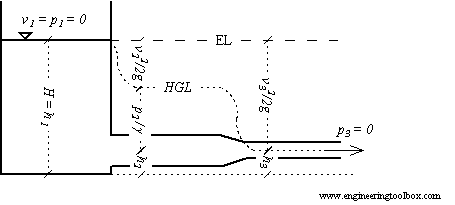Engineering ToolBox - Resources, Tools and Basic Information for Engineering and Design of Technical Applications!

# Energy and Hydraulic Grade Line

## The hydraulic grade line and the energy line are graphical presentations of the Bernoulli equation.

### The Bernoulli Equation

For steady, inviscid (having zero viscosity), incompressible flow the total energy remains constant along a stream line as expressed with the Bernoulli Equation:

p + 1/2 ρ v2 + γ h = constant along a streamline                            (1)

where

p = static pressure (relative to the moving fluid) (Pa, N/m2)

ρ = density (kg/m3)

γ = specific weight of fluid (N/m3)

v = flow velocity (m/s)

g = acceleration of gravity (9.81 m/s2)

h = elevation height (m)

Each term of this equation has the dimension force per unit area - N/m2 or psi, lb/ft2 in Imperial units.By dividing each term with the specific weight - γ = ρ g - equation (1) can be transformed to express head as

p / γ + v2 / 2 g + h = constant along a streamline = H                             (2)

where

H = the total head (m fluid column)

Each term of this equation has the dimension length - m or ft in Imperial units.

Note! - the head unit is with reference to the density of the flowing fluid. For other units - like mm Water Column - check Velocity Pressure Head.

(2) states that the sum of

• pressure head - p / γ
• velocity head - v2 / 2 g

is constant along a stream line. This constant can be called the total head - H.

The total head in a flow can be determined by measuring the stagnation pressure using a pitot tube.

The sum of pressure head - p / γ - and elevation head - h - is called the piezometric head. The piezometric head in a flow can be measured through an flat opening parallel to the flow.

### The Energy Line

The Energy Line is a line that represent the total head available to the fluid and can be expressed as:

EL = H = p / γ + v2 / 2 g + h = constant along a streamline                                 (3)

where

EL = Energy Line (m fluid column)

For a fluid flow without any losses due to friction (major losses) or components (minor losses) - the energy line would be at a constant level. In a practical world the energy line decreases along the flow due to losses.

A turbine in the flow reduces the energy line and a pump or fan in the line increases the energy line.

The Hydraulic Grade Line is a line representing the total head available to the fluid - minus the velocity head and can be expressed as:

HGL = p / γ + h                          (4)

where

HGL = Hydraulic Grade Line (m fluid column)

The hydraulic grade line lies one velocity head below the the energy line.

## Related Topics

• ### Fluid Mechanics

The study of fluids - liquids and gases. Involving velocity, pressure, density and temperature as functions of space and time.

## Related Documents

• ### Bernoulli Equation

Conservation of energy in a non-viscous, incompressible fluid at steady flow.
• ### Energy

Energy is the capacity to do work.
• ### Energy Equation - Pressure Loss vs. Head Loss

Calculate pressure loss - or head loss - in ducts, pipes or tubes.
• ### Pitot Tubes

Pitot tubes can be used to measure fluid flow velocities by measuring the difference between static and dynamic pressure in the flow.

Static pressure graphical presentation throughout a fluid flow system.
• ### U-Tube Differential Pressure Manometers

Inclined and vertical u-tube manometers used to measure differential pressure in flow meters like pitot tubes, orifices and nozzles.

## Engineering ToolBox - SketchUp Extension - Online 3D modeling!

Add standard and customized parametric components - like flange beams, lumbers, piping, stairs and more - to your Sketchup model with the Engineering ToolBox - SketchUp Extension - enabled for use with older versions of the amazing SketchUp Make and the newer "up to date" SketchUp Pro . Add the Engineering ToolBox extension to your SketchUp Make/Pro from the Extension Warehouse !

We don't collect information from our users. More about

## Citation

• The Engineering ToolBox (2003). Energy and Hydraulic Grade Line. [online] Available at: https://www.engineeringtoolbox.com/energy-hydraulic-grade-line-d_613.html [Accessed Day Month Year].

Modify the access date according your visit.

9.19.12